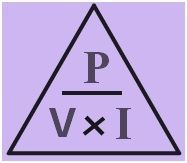# Power Formula

Power Formula

The capacity to do work is termed as Energy. The Energy expended to do work in unit time is termed as Power. It is represented as P.
Power Formula is articulated as,

or,

Where,
The Energy Consumed to do work = E
Work done = and
Time taken=

In any electrical circuit, the power is computed making use of these three formulasIn regards of Voltage and current, it is articulated as

In regards to current and resistance, it is articulated as

In regards to voltage and resistance, it is articulated as

Where,
Voltage applied across the two ends =V,

Current flowing in the circuit = I and
Resistance = R.

The Power Formula is made use of to compute the Power, Resistance, Voltage or current in any electrical circuit. It is articulated in watts.

Power Solved Examples

Underneath are some solved samples on Power which help comprehend these formulas.

Problem 1: An electric machine makes use of 300 J of energy to do work in 10s. How much power does it use?

Known: Work done = W = 300 J,
Time taken t = 10 s.

Problem 2: John is who has a mass of 60 kg runs up to 12m high in 40 seconds. Compute his power.